# Samacheer Kalvi 8th Maths Solutions Term 1 Chapter 5 Information Processing Intext Questions

Students can Download Maths Chapter 5 Information Processing Intext Questions and Answers, Notes Pdf, Samacheer Kalvi 8th Maths Book Solutions Guide Pdf helps you to revise the complete Tamilnadu State Board New Syllabus and score more marks in your examinations.## Tamilnadu Samacheer Kalvi 8th Maths Solutions Term 1 Chapter 5 Information Processing Intext Questions

Try these (TextBook Page No 122)

Question 1.
Find the number of all possible triangles that can be formed from the triangle given below.
Solution: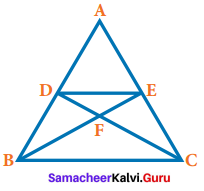Question 2.
Use the given figure to form a 3 × 3 magic square.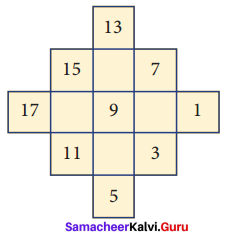Solution: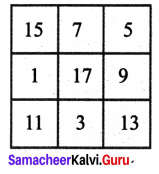Question 3.
Convert the tree diagram into a numeric expression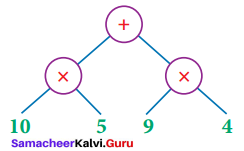Solution:
(10 × 5) + (9 × 4)

Question 4.
(i) Find out the total time taken by the bus to reach from A to E via B , C and D.
(ii) Find which is the shortest route from A to E.
Solution: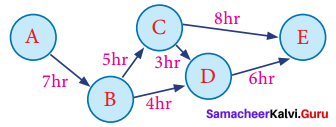(i) Route ⇒ 2A ➝ B ➝ C ➝ D ➝ E
Time taken ⇒ (7 + 5 + 3 + 6) hrs = 21 hrs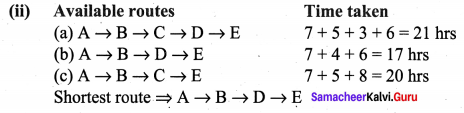Try this (TextBook Page No 127)

Question 1.
Kumar has four different hats. He always wears a hat. Sometimes he wears the same hat more than once in a week as in the figure.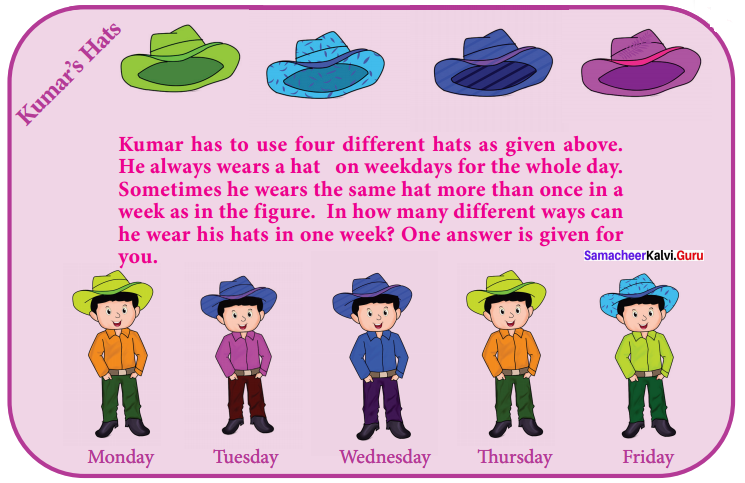In how many different ways might he decide to wear his hats in one week?
Solution: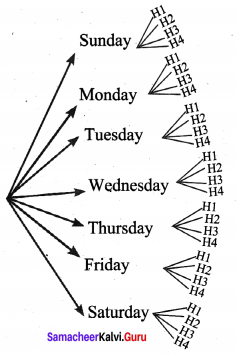H1, H2, H3, H4 be Hat 1, Hat 2, Hat 3, Hat 4 respectively.
∴ In 7 × 4 = 28 different ways kumar may decide to wear his hat in one week.

Exercise 5.1

Try these (TextBook Page No 134)

Question 1.
Find any four SETs among these set of cards.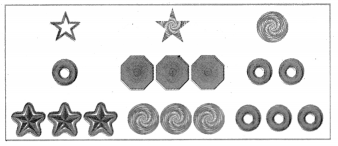Solution: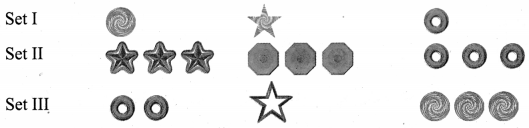Question 2.
This is an example for a magic square in SETs, can you make another one?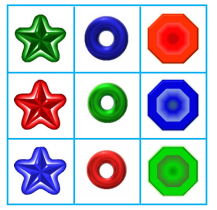Solution: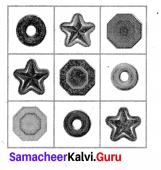Try this (TextBook PageNo 137)

Question 1.
Think why this graph colouring is invalid?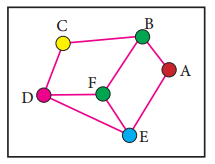Solution:
The objective of graph colouring is to assign minimum number of colours to the verties do not have the same colour.
Here adjacent edges have the same colour.
∴ It is invalid.